# How do I correct or clear an equation?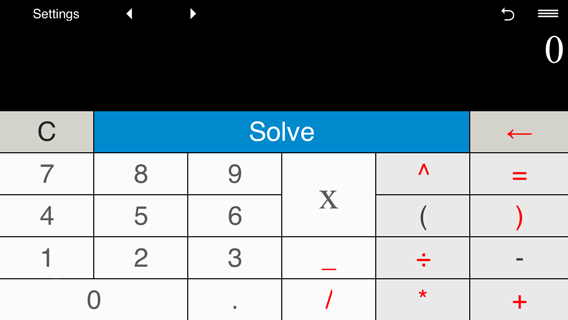In the Advanced mode, to delete the last character in the displayed equation, tap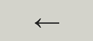.

To clear the calculation, tap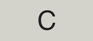#### Correcting previous equations.

xSolver allows you to recall previous equations from the Calculation history.

Use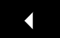and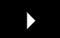to browse the Calculation history.

To correct an equation, display it usingand, input the correct values and tap "Solve".

### Built-in formats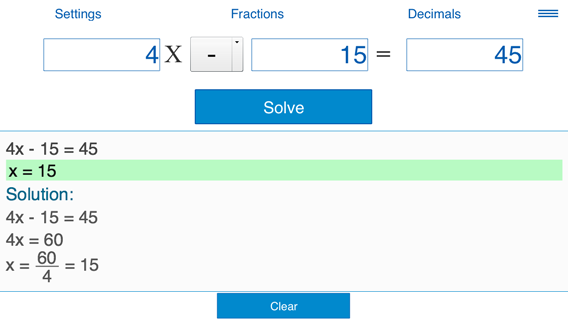To edit the displayed equation, just enter the correct values of coefficients.

To clear the displayed equation, tap Clear.

#### Correcting previous equations.

To edit an equation that was entered in the built-in templates "ax + b = c" and "ax + b = cx + d", switch to the Advanced format, recall the equations usingandand make the necessary changes.